Anúncio

# To Study the earth's magnetic field using a tangent galvanometer Tangent galvanometer

24 de Jan de 2018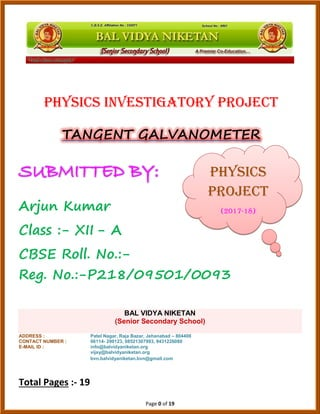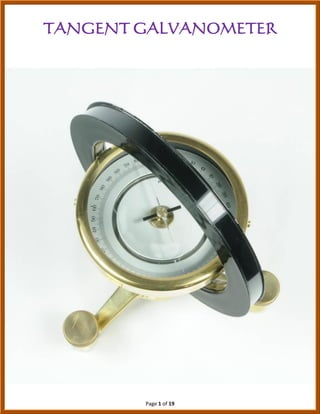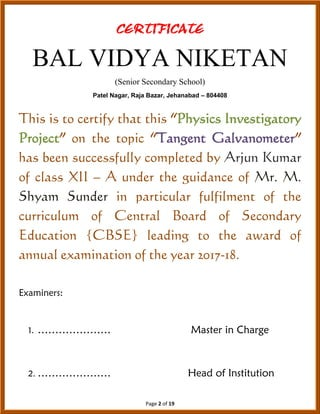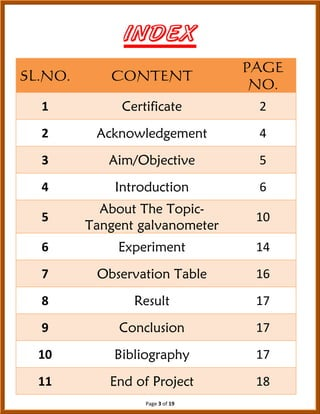Anúncio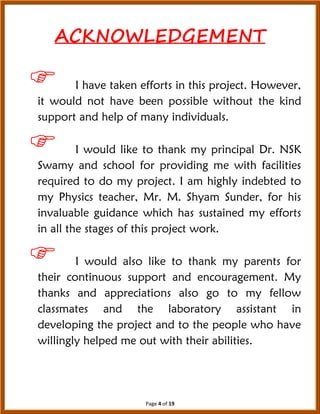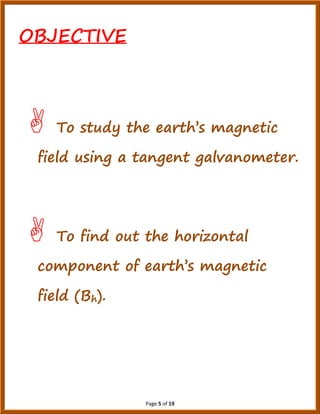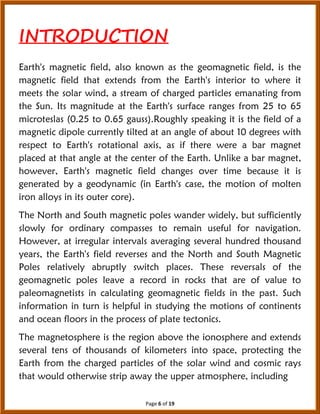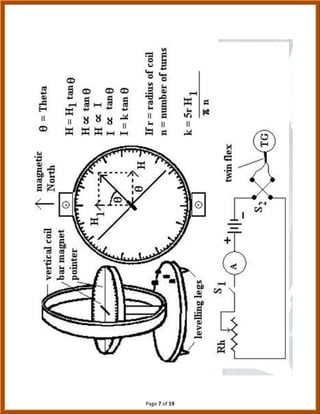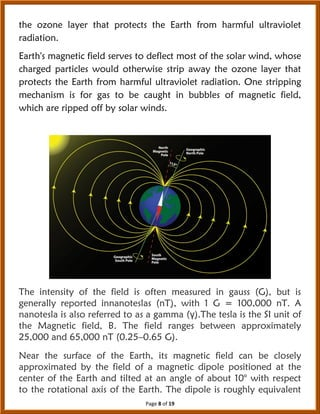Anúncio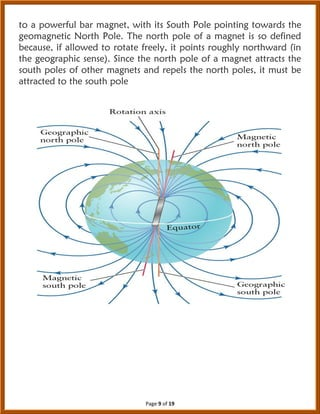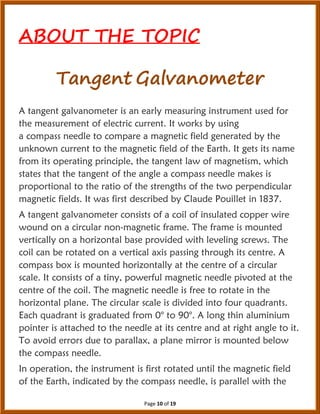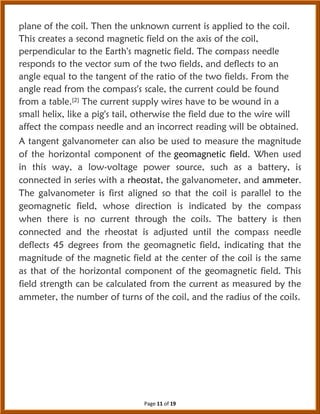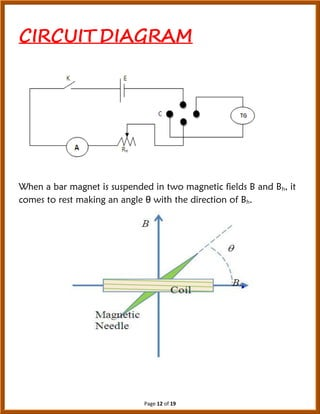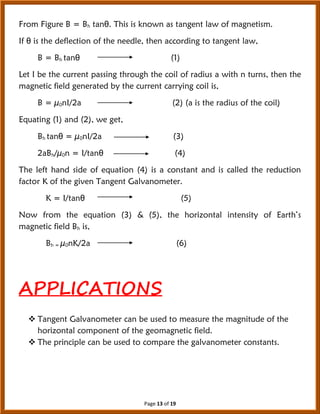Anúncio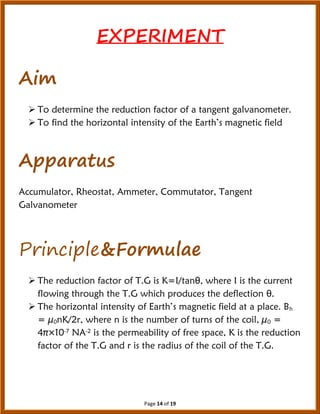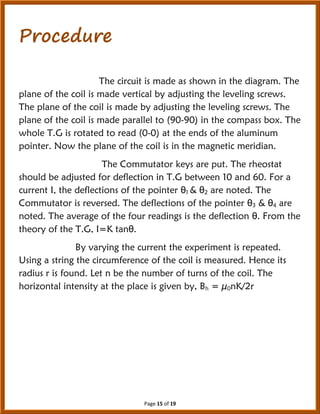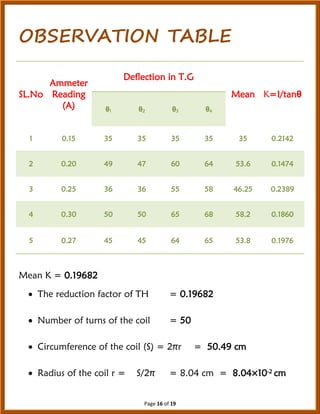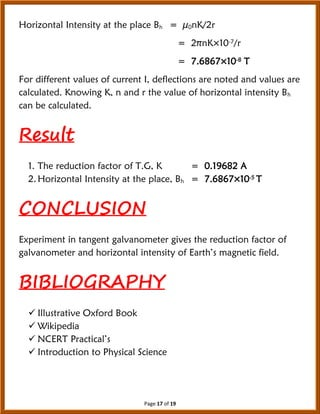Próximos SlideShares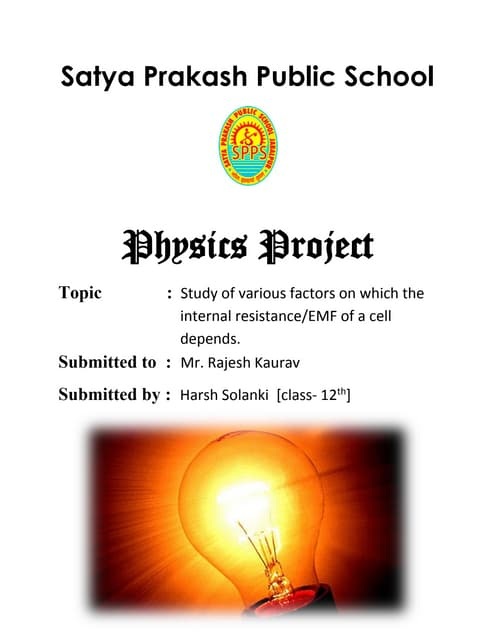Factors on which the internal resistance/emf of a cell depends
Carregando em ... 3
1 de 19
Anúncio

### To Study the earth's magnetic field using a tangent galvanometer Tangent galvanometer

1. Page 0 of 19 PHYSICS INVESTIGATORY PROJECT TANGENT GALVANOMETER SUBMITTED BY: Arjun Kumar Class :- XII - A CBSE Roll. No.:- Reg. No.:-P218/09501/0093 BAL VIDYA NIKETAN (Senior Secondary School) ADDRESS : Patel Nagar, Raja Bazar, Jehanabad – 804408 CONTACT NUMBER : 06114- 290123, 08521307993, 9431226080 E-MAIL ID : info@balvidyaniketan.org vijay@balvidyaniketan.org bvn.balvidyaniketan.bvn@gmail.com Total Pages :- 19 PHYSICS Project (2017-18)
2. Page 1 of 19 TANGENT GALVANOMETER
3. Page 2 of 19 BAL VIDYA NIKETAN (Senior Secondary School) Patel Nagar, Raja Bazar, Jehanabad – 804408 This is to certify that this “Physics Investigatory Project” on the topic “Tangent Galvanometer” has been successfully completed by Arjun Kumar of class XII – A under the guidance of Mr. M. Shyam Sunder in particular fulfilment of the curriculum of Central Board of Secondary Education {CBSE} leading to the award of annual examination of the year 2017-18. Examiners: 1. ………………… Master in Charge 2. ………………… Head of Institution
4. Page 3 of 19 SL.NO. CONTENT PAGE NO. 1 Certificate 2 2 Acknowledgement 4 3 Aim/Objective 5 4 Introduction 6 5 About The Topic- Tangent galvanometer 10 6 Experiment 14 7 Observation Table 16 8 Result 17 9 Conclusion 17 10 Bibliography 17 11 End of Project 18
5. Page 4 of 19 ACKNOWLEDGEMENT  I have taken efforts in this project. However, it would not have been possible without the kind support and help of many individuals.  I would like to thank my principal Dr. NSK Swamy and school for providing me with facilities required to do my project. I am highly indebted to my Physics teacher, Mr. M. Shyam Sunder, for his invaluable guidance which has sustained my efforts in all the stages of this project work.  I would also like to thank my parents for their continuous support and encouragement. My thanks and appreciations also go to my fellow classmates and the laboratory assistant in developing the project and to the people who have willingly helped me out with their abilities.
6. Page 5 of 19 OBJECTIVE  To study the earth’s magnetic field using a tangent galvanometer.  To find out the horizontal component of earth’s magnetic field (Bh).
7. Page 6 of 19 INTRODUCTION Earth's magnetic field, also known as the geomagnetic field, is the magnetic field that extends from the Earth's interior to where it meets the solar wind, a stream of charged particles emanating from the Sun. Its magnitude at the Earth's surface ranges from 25 to 65 microteslas (0.25 to 0.65 gauss).Roughly speaking it is the field of a magnetic dipole currently tilted at an angle of about 10 degrees with respect to Earth's rotational axis, as if there were a bar magnet placed at that angle at the center of the Earth. Unlike a bar magnet, however, Earth's magnetic field changes over time because it is generated by a geodynamic (in Earth's case, the motion of molten iron alloys in its outer core). The North and South magnetic poles wander widely, but sufficiently slowly for ordinary compasses to remain useful for navigation. However, at irregular intervals averaging several hundred thousand years, the Earth's field reverses and the North and South Magnetic Poles relatively abruptly switch places. These reversals of the geomagnetic poles leave a record in rocks that are of value to paleomagnetists in calculating geomagnetic fields in the past. Such information in turn is helpful in studying the motions of continents and ocean floors in the process of plate tectonics. The magnetosphere is the region above the ionosphere and extends several tens of thousands of kilometers into space, protecting the Earth from the charged particles of the solar wind and cosmic rays that would otherwise strip away the upper atmosphere, including
8. Page 7 of 19
9. Page 8 of 19 the ozone layer that protects the Earth from harmful ultraviolet radiation. Earth's magnetic field serves to deflect most of the solar wind, whose charged particles would otherwise strip away the ozone layer that protects the Earth from harmful ultraviolet radiation. One stripping mechanism is for gas to be caught in bubbles of magnetic field, which are ripped off by solar winds. The intensity of the field is often measured in gauss (G), but is generally reported innanoteslas (nT), with 1 G = 100,000 nT. A nanotesla is also referred to as a gamma (γ).The tesla is the SI unit of the Magnetic field, B. The field ranges between approximately 25,000 and 65,000 nT (0.25–0.65 G). Near the surface of the Earth, its magnetic field can be closely approximated by the field of a magnetic dipole positioned at the center of the Earth and tilted at an angle of about 10° with respect to the rotational axis of the Earth. The dipole is roughly equivalent
10. Page 9 of 19 to a powerful bar magnet, with its South Pole pointing towards the geomagnetic North Pole. The north pole of a magnet is so defined because, if allowed to rotate freely, it points roughly northward (in the geographic sense). Since the north pole of a magnet attracts the south poles of other magnets and repels the north poles, it must be attracted to the south pole
11. Page 10 of 19 ABOUT THE TOPIC Tangent Galvanometer A tangent galvanometer is an early measuring instrument used for the measurement of electric current. It works by using a compass needle to compare a magnetic field generated by the unknown current to the magnetic field of the Earth. It gets its name from its operating principle, the tangent law of magnetism, which states that the tangent of the angle a compass needle makes is proportional to the ratio of the strengths of the two perpendicular magnetic fields. It was first described by Claude Pouillet in 1837. A tangent galvanometer consists of a coil of insulated copper wire wound on a circular non-magnetic frame. The frame is mounted vertically on a horizontal base provided with leveling screws. The coil can be rotated on a vertical axis passing through its centre. A compass box is mounted horizontally at the centre of a circular scale. It consists of a tiny, powerful magnetic needle pivoted at the centre of the coil. The magnetic needle is free to rotate in the horizontal plane. The circular scale is divided into four quadrants. Each quadrant is graduated from 0° to 90°. A long thin aluminium pointer is attached to the needle at its centre and at right angle to it. To avoid errors due to parallax, a plane mirror is mounted below the compass needle. In operation, the instrument is first rotated until the magnetic field of the Earth, indicated by the compass needle, is parallel with the
12. Page 11 of 19 plane of the coil. Then the unknown current is applied to the coil. This creates a second magnetic field on the axis of the coil, perpendicular to the Earth's magnetic field. The compass needle responds to the vector sum of the two fields, and deflects to an angle equal to the tangent of the ratio of the two fields. From the angle read from the compass's scale, the current could be found from a table. The current supply wires have to be wound in a small helix, like a pig's tail, otherwise the field due to the wire will affect the compass needle and an incorrect reading will be obtained. A tangent galvanometer can also be used to measure the magnitude of the horizontal component of the geomagnetic field. When used in this way, a low-voltage power source, such as a battery, is connected in series with a rheostat, the galvanometer, and ammeter. The galvanometer is first aligned so that the coil is parallel to the geomagnetic field, whose direction is indicated by the compass when there is no current through the coils. The battery is then connected and the rheostat is adjusted until the compass needle deflects 45 degrees from the geomagnetic field, indicating that the magnitude of the magnetic field at the center of the coil is the same as that of the horizontal component of the geomagnetic field. This field strength can be calculated from the current as measured by the ammeter, the number of turns of the coil, and the radius of the coils.
13. Page 12 of 19 CIRCUIT DIAGRAM When a bar magnet is suspended in two magnetic fields B and Bh, it comes to rest making an angle θ with the direction of Bh.
14. Page 13 of 19 From Figure B = Bh tanθ. This is known as tangent law of magnetism. If θ is the deflection of the needle, then according to tangent law, B = Bh tanθ (1) Let I be the current passing through the coil of radius a with n turns, then the magnetic field generated by the current carrying coil is, B = µ0nI/2a (2) (a is the radius of the coil) Equating (1) and (2), we get, Bh tanθ = µ0nI/2a (3) 2aBh/µ0n = I/tanθ (4) The left hand side of equation (4) is a constant and is called the reduction factor K of the given Tangent Galvanometer. K = I/tanθ (5) Now from the equation (3) & (5), the horizontal intensity of Earth’s magnetic field Bh is, Bh = µ0nK/2a (6) APPLICATIONS  Tangent Galvanometer can be used to measure the magnitude of the horizontal component of the geomagnetic field.  The principle can be used to compare the galvanometer constants.
15. Page 14 of 19 EXPERIMENT Aim  To determine the reduction factor of a tangent galvanometer.  To find the horizontal intensity of the Earth’s magnetic field Apparatus Accumulator, Rheostat, Ammeter, Commutator, Tangent Galvanometer Principle&Formulae  The reduction factor of T.G is K=I/tanθ, where I is the current flowing through the T.G which produces the deflection θ.  The horizontal intensity of Earth’s magnetic field at a place. Bh = µ0nK/2r, where n is the number of turns of the coil, µ0 = 4π×10-7 NA-2 is the permeability of free space, K is the reduction factor of the T.G and r is the radius of the coil of the T.G.
16. Page 15 of 19 Procedure The circuit is made as shown in the diagram. The plane of the coil is made vertical by adjusting the leveling screws. The plane of the coil is made by adjusting the leveling screws. The plane of the coil is made parallel to (90-90) in the compass box. The whole T.G is rotated to read (0-0) at the ends of the aluminum pointer. Now the plane of the coil is in the magnetic meridian. The Commutator keys are put. The rheostat should be adjusted for deflection in T.G between 10 and 60. For a current I, the deflections of the pointer θ1 & θ2 are noted. The Commutator is reversed. The deflections of the pointer θ3 & θ4 are noted. The average of the four readings is the deflection θ. From the theory of the T.G, I=K tanθ. By varying the current the experiment is repeated. Using a string the circumference of the coil is measured. Hence its radius r is found. Let n be the number of turns of the coil. The horizontal intensity at the place is given by, Bh = µ0nK/2r
17. Page 16 of 19 OBSERVATION TABLE SL.No Ammeter Reading (A) Deflection in T.G Mean K=I/tanθ θ1 θ2 θ3 θ4 1 0.15 35 35 35 35 35 0.2142 2 0.20 49 47 60 64 53.6 0.1474 3 0.25 36 36 55 58 46.25 0.2389 4 0.30 50 50 65 68 58.2 0.1860 5 0.27 45 45 64 65 53.8 0.1976 Mean K = 0.19682  The reduction factor of TH = 0.19682  Number of turns of the coil = 50  Circumference of the coil (S) = 2πr = 50.49 cm  Radius of the coil r = S/2π = 8.04 cm = 8.04×10-2 cm
18. Page 17 of 19 Horizontal Intensity at the place Bh = µ0nK/2r = 2πnK×10-7/r = 7.6867×10-8 T For different values of current I, deflections are noted and values are calculated. Knowing K, n and r the value of horizontal intensity Bh can be calculated. Result 1. The reduction factor of T.G, K = 0.19682 A 2.Horizontal Intensity at the place, Bh = 7.6867×10-5 T CONCLUSION Experiment in tangent galvanometer gives the reduction factor of galvanometer and horizontal intensity of Earth’s magnetic field. BIBLIOGRAPHY  Illustrative Oxford Book  Wikipedia  NCERT Practical’s  Introduction to Physical Science
19. Page 18 of 19 End of Project Thank you!
Anúncio